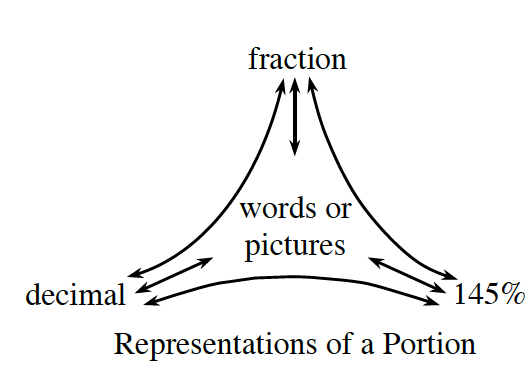# testaaa

HAM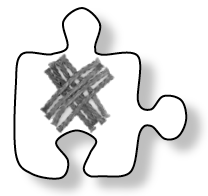# 6.1.4How does it make sense?

Solving Problems Involving Fraction Division

In Lesson 6.1.3, you made sense of the answers to division problems.  You paid particular attention to the meaning of each part of the division sentence.  In this lesson, you will extend your understanding about dividing to include division of fractions by other fractions.  As you work with your team, recall what you know about the relationship between multiplication and division and keep the following questions in mind.

How can we represent this problem with a diagram?

Can we represent it in more than one way?

6-43.

Dria is writing a piece of music.  She has decided to replace a

$34$

note which takes up

$34$

of a small section of the music called a measure, with

$18$

notes which each take up

$18$

of a measure.  Work with your team to use diagrams to help you figure out how many

$18$

notes she will need.  Then represent the problem and its solution with a mathematical division sentence and a diagram.  Be prepared to describe your strategies to the class.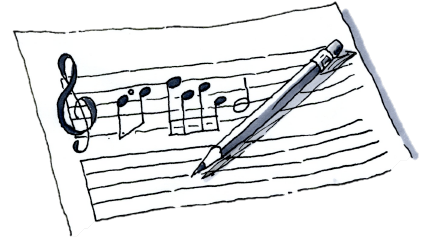6-44.

Malik was catching up on homework when he noticed that he got the same answer dividing

$3$

by

$15$

as he did when he multiplied

$3$

by

$5$

.

In other words,

$3÷15=3·5=15$

.  He asked his teammates, “Is dividing by

$15$

always the same as multiplying by 5?”

1. Liam drew the two diagrams below and wrote down

and

$1÷15=5$

.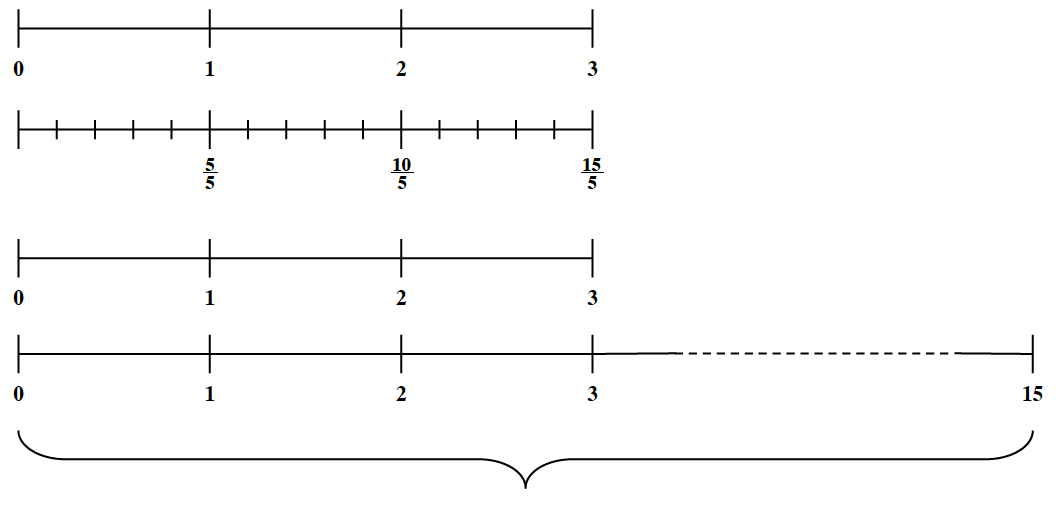Does it have something to do with the fact that there are

$5$

one-fifths in each whole,

$10$

one-fifths in

$2$

wholes and

$15$

one-fifths in

$3$

“Or,” asked Malik, “Is it because

$1$

is

$15$

of

$5$

$2$

is

$15$

of

$10$

and

$3$

is

$15$

of

$15$

?”

Discuss this with your team.  Do Liam’s and Malik’s explanations both make sense?  Why or why not?  Can you think of any other ways to explain this?

1. Malik was looking at problem 6-43 and asked, “Does this work when both numbers are fractions?  Can you find how many

$18$

s are in

$34$

by multiplying

$34$

by

$8$

?”  What do you think?  Refer back to the diagram you drew for problem 6-43.  Be ready to explain your ideas.

6-45.

DORA’S DOLLHOUSE, Part 1

Dora is building a dollhouse for her cousin.  She needs several boards that are each

$34$

of a foot long.  She went to the store and found that the lumber she needs is sold only in lengths of

$8$

feet.  She laid a tape measure next to a board and drew the diagram below.  The diagram is also available on the Lesson 6.1.4 Resource Page, “Dora’s Dollhouse”.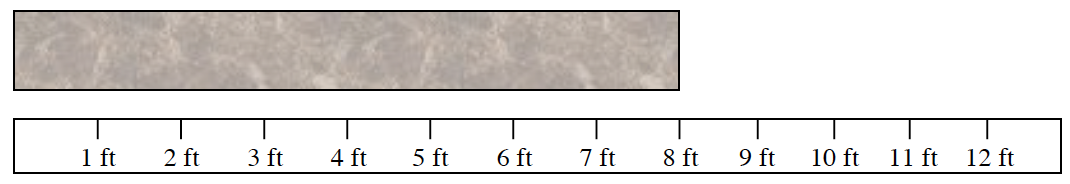1. Work with your team to figure out how many of her

$34$

-foot boards she can cut from one

$8$

-foot piece of wood.  Be prepared to explain how you got your answer and to show why it makes sense using the diagram.

2. After she cuts her boards, how much lumber will be left over from the

$8$

foot piece?  What part of a

$34$

foot board is this?  Show how you know.

3. Represent this situation with a division sentence.

6-46.

DORA’S DOLLHOUSE, Part 2

Dora has taken a closer look at her blueprints and figured out that one

$8$

foot board is exactly

$34$

of the length of wood that she needs for her whole project.

1. What length of wood does she need for her project?  Work with your team to use the second diagram on the Lesson 6.1.4 Resource Page, “Dora’s Dollhouse,” to help you make sense of this question.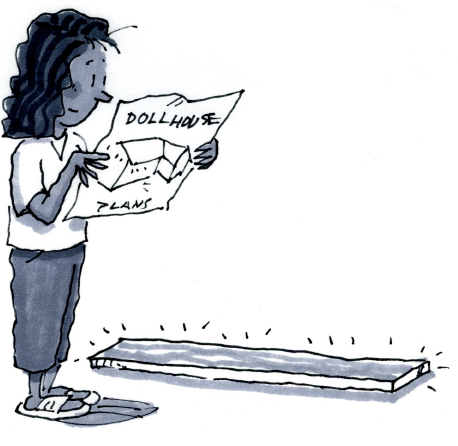1. Represent this question and its answer with division.

2. Compare this problem with the question and answer in problem 6-45.  In what ways are these questions and answers the same?  How are they different?

6-47.

DIVISION AND AREA

The Ferndale High School Golden Eagles have a large playfield at their school that covers

$23$

of a square mile.  One side of the playfield measures

$12$

mile long.

1. Draw a diagram that shows this situation.  Be sure to include labels.

2. What operation can you do to calculate the other dimension of the field? Write a number sentence for this operation.

3. What is the other dimension of the playing field?What strategy did you use?

6-48.

TEAM DIVISION

For each of the division problems below, work with your team to:

• Write a question in words that could be answered using the expression.

• Draw a diagram that represents the problem and your question.

• Find the quotient and explain what it means.

1. $12÷34$

6-49.

You may have noticed that one answer (quotient) in problem 6-48 is greater than one and that one of the answers is less than one, even though all of the numbers in the problem are less than one.

1. Work with your team to figure out why this makes sense.  Be ready to explain your thinking.

2. Write and solve two new fraction division problems.  Write one that has an answer less than one and another that has an answer greater than one.  Be ready to share your problems with the class.

6-50.

TEAM LEARNING LOG

In previous chapters, you explored the effects of multiplication.  You enlarged and reduced figures by multiplying by numbers that were less than or greater than one.  Today you will write a team Learning Log that describes the effects of division. With your team, discuss the following questions:With your team, discuss the following questions:

Do division problems always result in an answer that is less
than or greater than the original number?

How does the size of the divisor (the number you are

Can the answer to a division problem be greater than the
original number?

Once you have agreed on the answers, write your conclusions in the form of a statement that you all agree is true about the effects of division.  Then use your team statement to begin your own individual Leaning Log, but add your own examples to support and justify it.  Title this Learning Log “The Effects of Division” and label it with today’s date.6-51.

Name the coordinates of each point shown in the graph at right using ordered pairs

$(x,y)$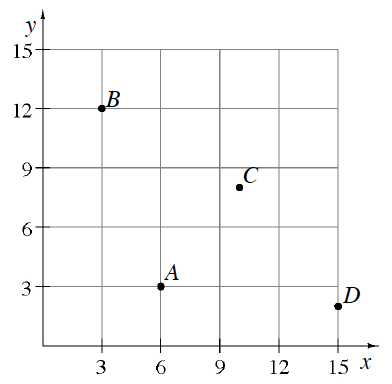6-52.

At the school’s fall bake sale, all of the pies were cut into 6 pieces, so each person who bought a piece bought

$16$

of a pie. Each slice of pie sold for

$1.00$

. How much money did the school make if all eleven pies were sold? (In other words, find

$11$

divided by

$16$
6-53.

Find the area and perimeter of a rectangle that is

$14.5$

meters by

$5.8$

meters. Homework Help ✎

6-54.

Express each of the following numbers as a product of its prime factors. Use exponents to represent repeated multiplication, when applicable. An example is given below. Homework Help ✎

$40=2·20=2·2·10=2·2·2·5=23·5$

1. $30$

1. $300$

1. $17$

1. $21$

6-55.

Copy the pattern below. Homework Help ✎

 $∙$ Figure 1 $∙∙∙∙$ Figure 2 $∙∙∙∙∙∙∙$ Figure 3 $∙∙∙∙∙∙∙∙∙∙$ Figure 4
1. Draw the fifth and sixth figures on your paper.

2. Describe Figure 20.

3. How many dots will be in Figure 20?

6-56.

Graph each of the following points and connect them in order. 6-56 HW eTool (Desmos)   Homework Help ✎

$(1,1),(1,5),(5,5),(5,1),(3,1),(3,3),(2,3),(2,1),(1,1)$

1. What is the area of the shape that was formed?

2. What is the perimeter of the shape that was formed?

6-57.

Ms. Perez is giving her class a pizza party because every student completed the school‑wide book reading challenge. If an extra-large pizza costs

$15$

and serves

$8$

people, how much should Ms. Perez expect to pay for pizzas if her class has

$28$

students? Homework Help ✎

6-58.

(total length)(total width) = sum of individual area parts = total area. Homework Help ✎

1.  $+6$ $20$ $1600$ $560$
1.  $+5$ $30$ $2700$ $3600$ $45$
6-59.

In each of these problems, assume that people divide the food evenly. Write your answer as a division problem in fraction form:

$numbernumber$
1. If two people share one soda, how much of the soda should each person get?

2. If two people share three hamburgers, how much should each person get?

3. If three people share a large box of fries, what part is each person’s share?

4. Three people share seven brownies. How many brownies should each person get?

5. Two people share five apple turnovers. How many turnovers should each person get?

6. If five people share three cartons of chow mein, what is each person’s share?

6-60.

Complete the web shown below to represent the portion

$145%$

as a fraction, a decimal, and with words. Homework Help ✎